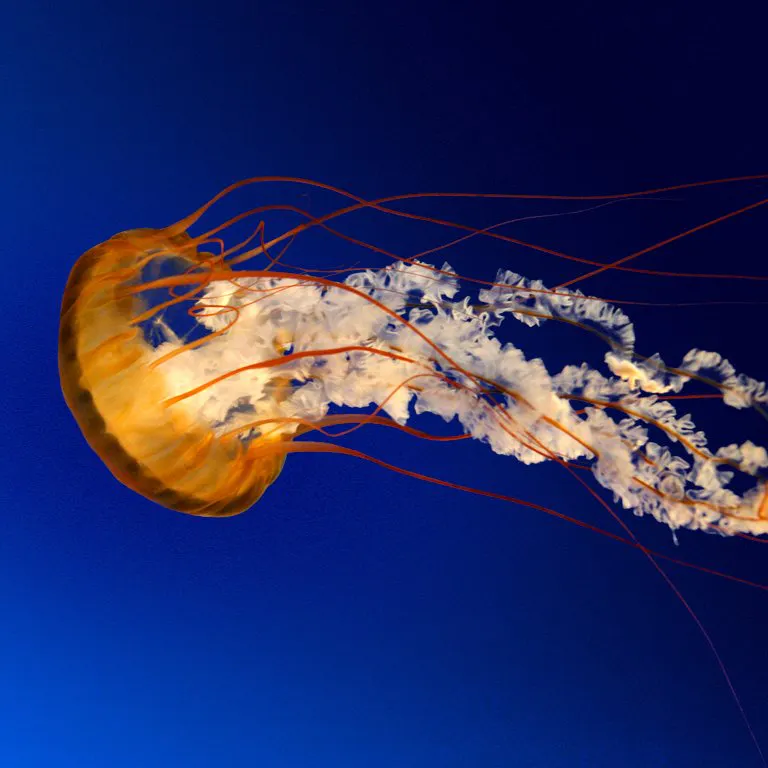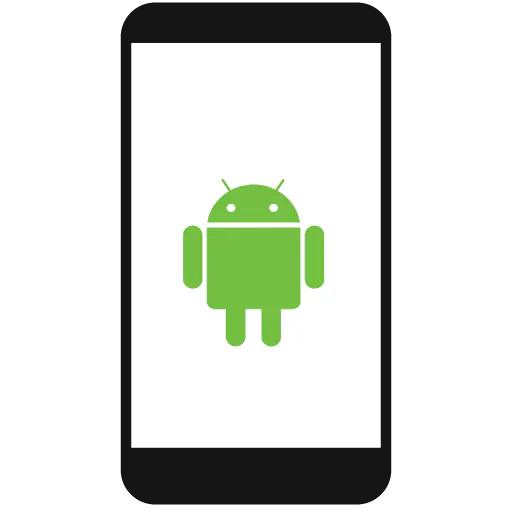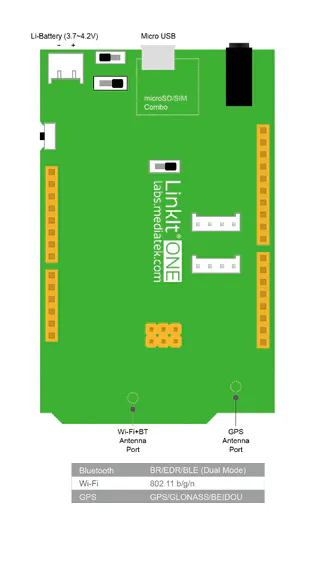Published

# Parked Car Finder

Using a Linkit One, I send GPS data to my phone so I never forget where I parked my car!

BeginnerFull instructions provided1,246## Things used in this project

### Hardware componentsAndroid device
×1

Read more

## Schematics

### Linkit One Connections

You only need the Linkit One, Bluetooth antenna, GPS antenna and Battery (all of which came with the Linkit One box)## Code

### BluetoothWithGPS

Arduino
The code I use to get the Linkit One to send me information. It relies on a couple of libraries (that come when you setup the Linkit One)
```// Libraries that I use
#include <LGPS.h>
#include <LBT.h>
#include <LBTServer.h>

// Create the GPS sentence structure for the GPS component
gpsSentenceInfoStruct info;

// Create the buff variable for the bluetooth component
char buff;

// getComma is from the GPS example to seperate the GPS string
static unsigned char getComma(unsigned char num,const char *str)
{
unsigned char i,j = 0;
int len=strlen(str);
for(i = 0;i < len;i ++)
{
if(str[i] == ',')
j++;
if(j == num)
return i + 1;
}
return 0;
}

// getDoubleNumber is from the GPS example to convert the string to a number
static double getDoubleNumber(const char *s)
{
char buf;
unsigned char i;
double rev;

i=getComma(1, s);
i = i - 1;
strncpy(buf, s, i);
buf[i] = 0;
rev=atof(buf);
return rev;
}

// getIntNumber is from the GPS example to convert the string to a number
static double getIntNumber(const char *s)
{
char buf;
unsigned char i;
double rev;

i=getComma(1, s);
i = i - 1;
strncpy(buf, s, i);
buf[i] = 0;
rev=atoi(buf);
return rev;
}

// I start my setup section
void setup() {
// I setup my baud rate at 9600
Serial.begin(9600);
// I turn on the GPS section of the Linkit One
LGPS.powerOn();
// Tell the serial port that it is on.
Serial.println("LGPS Power on, and waiting ...");

delay(3000);

// I startup the bluetooth component and tell the serial port it's working
if(!LBTServer.begin((uint8_t*)"My_BTServer"))
{
Serial.println("Fail to start BT.");
return;
}
Serial.println("BT server is started.");
}

void loop() {
// put your main code here, to run repeatedly:
Serial.println("LGPS loop");
// I grab the GPS data
LGPS.getData(&info);
// I set the variable GPGGAstr to be the info
const char* GPGGAstr = (const char*)info.GPGGA;

// I create the variable lat and lon (to get the all the digits)
double lat = 0;
double lon = 0;
// I create the variables latitude and longitude to convert lat and lon
// and use those string values
String latitude;
String longitude;

// buf and bytesRead is used for the bluetooth portion
uint8_t buf;
int bytesRead;

int tmp;

// If something connects to the Linkit One via bluetooth then I can start
// sending information to the phone
if(LBTServer.connected())
{
// if the start of the GPS string is a \$ sign then I know that I grabbed
// it correctly
if(GPGGAstr == '\$')
{
// I set the temporary variable to be the latitude section
tmp = getComma(2, GPGGAstr);
// I use the getDoubleNumber to convert that string to a double
lat = getDoubleNumber(&GPGGAstr[tmp]);
// I set the temporary variable to be the longitude section
tmp = getComma(4, GPGGAstr);
// I use the getDoubleNumber to convert that string to a double
lon = getDoubleNumber(&GPGGAstr[tmp]);

// I don't need these after I verify it. The String() function
// can be used to specify the number of digits (in this case 6)
//Serial.println(String(lat/100,6));
//Serial.println(String(lon/100,6));

// I set the latitude to have 6 digits in the decimal place and
// at a comma at the end so I can interpret it correctly
latitude = String(lat/100,6) + ',';
// I set the longitude to have 6 digits in the decimal place
// and add a negative sign at the beginning (because the GPS
// coordinate doesn't have the negative sign built in
longitude = '-' + String(lon/100,6);

// I set strlen1 to be the size of the latitude string +1
int strlen1 = latitude.length() + 1;
// I set strlen2 to be the size of the longitude string +1
int strlen2 = longitude.length() + 1;
// I use bufspot for bytesRead portion (bytesRead is the verification
// that the transmission sent correctly)
int bufspot = 0;
// I create two arrays to fill in the buf array
char chararray1[strlen1];
char chararray2[strlen2];
// I use the function toCharArray() to fill in the two arrays I just
// created.
latitude.toCharArray(chararray1,strlen1);
longitude.toCharArray(chararray2,strlen2);

// I create the variable i so that I can use it for the for loop
int i;

// I start filling out the buf array for the bluetooth transmission
// I increase the variable bufspot each time I loop
for (i = 0; i<strlen1; i++) {
buf[i] = chararray1[i];
bufspot++;
}

// I set the variable buf2 to the value of bufspot
int buf2 = bufspot;

// I iterate from bufspot to bufspot+strlen2 and I fill
// out the buf array with the remaining information. I
// increase buf2 each time (which will be used to set
// bytesRead to)
for (i = bufspot; i < bufspot+strlen2; i++) {
buf[i] = chararray2[i-bufspot];
buf2++;
}

// The last index of the buf array has to be 0 so that the
// transmission knows when to end
buf[buf2+1] = 0;
// I set bytesRead to buf2
bytesRead = buf2;

// This is an example of what the individual arrays look like
//buf = 'a';
//buf = 'b';
//buf = 'c';
//buf = 0;
//bytesRead = 4;

// I write the the serial port so that you can see what's going
// to be transmitted
Serial.write(buf, bytesRead);
// Then I start to transmit to the Server (in this case my phone)
// the same thing I sent to the Serial port
LBTServer.write(buf, bytesRead);

// This is all mostly unneccessary because this is the portion where
// I read back the transmissions from the phone but I will explain it
// anyway

// while(true) indicates whether or not I've correctly sent the information
// to the device
while(true)
{
// I set bytesRead to be whatever buf is at the moment (basically
// grabbing the transmission)
bytesRead = LBTServer.readBytes(buf, 64);

// I print back what I read to the Serial port
Serial.println(bytesRead);

// if I listen to it wrong then I want to break this code and
// move on
if(!bytesRead)
break;

// If the first letter that I read in is a capital S then I'm going
// to set the buf array with abc
if (buf == 'S') {
buf = 'a';
buf = 'b';
buf = 'c';
buf = 0;
bytesRead = 4;
// I'm then going to write to the serial port what I just put
// together
Serial.write(buf, bytesRead);
// And then I'm going to send it to the bluetooth connected
// device (in this case my phone)
LBTServer.write(buf, bytesRead);
}

} // end of the while(true) portion

// I wait 2 seconds before I can continue looping
delay(2000);

}
// If it doesn't have a \$ sign at the beginning then the GPS is not setup
// correctly
else
{
// I print out that it didn't get received correctly
Serial.println("Not get data");
}

} // end of the if(LBTServer.connected()) section

// If the bluetooth is not connected then I want to wait and listen
// for another connection. If I get one then I will be connected
else
{
LBTServer.accept(5);
}

} // end of loop() section
```

### GPS example

Arduino
This is the one that comes when you setup the Linkit One files.
```#include <LGPS.h>

gpsSentenceInfoStruct info;
char buff;

static unsigned char getComma(unsigned char num,const char *str)
{
unsigned char i,j = 0;
int len=strlen(str);
for(i = 0;i < len;i ++)
{
if(str[i] == ',')
j++;
if(j == num)
return i + 1;
}
return 0;
}

static double getDoubleNumber(const char *s)
{
char buf;
unsigned char i;
double rev;

i=getComma(1, s);
i = i - 1;
strncpy(buf, s, i);
buf[i] = 0;
rev=atof(buf);
return rev;
}

static double getIntNumber(const char *s)
{
char buf;
unsigned char i;
double rev;

i=getComma(1, s);
i = i - 1;
strncpy(buf, s, i);
buf[i] = 0;
rev=atoi(buf);
return rev;
}

void parseGPGGA(const char* GPGGAstr)
{
/* Refer to http://www.gpsinformation.org/dale/nmea.htm#GGA
* Sample data: \$GPGGA,123519,4807.038,N,01131.000,E,1,08,0.9,545.4,M,46.9,M,,*47
* Where:
*  GGA          Global Positioning System Fix Data
*  123519       Fix taken at 12:35:19 UTC
*  4807.038,N   Latitude 48 deg 07.038' N
*  01131.000,E  Longitude 11 deg 31.000' E
*  1            Fix quality: 0 = invalid
*                            1 = GPS fix (SPS)
*                            2 = DGPS fix
*                            3 = PPS fix
*                            4 = Real Time Kinematic
*                            5 = Float RTK
*                            6 = estimated (dead reckoning) (2.3 feature)
*                            7 = Manual input mode
*                            8 = Simulation mode
*  08           Number of satellites being tracked
*  0.9          Horizontal dilution of position
*  545.4,M      Altitude, Meters, above mean sea level
*  46.9,M       Height of geoid (mean sea level) above WGS84
*                   ellipsoid
*  (empty field) time in seconds since last DGPS update
*  (empty field) DGPS station ID number
*  *47          the checksum data, always begins with *
*/
double latitude;
double longitude;
int tmp, hour, minute, second, num ;
if(GPGGAstr == '\$')
{
tmp = getComma(1, GPGGAstr);
hour     = (GPGGAstr[tmp + 0] - '0') * 10 + (GPGGAstr[tmp + 1] - '0');
minute   = (GPGGAstr[tmp + 2] - '0') * 10 + (GPGGAstr[tmp + 3] - '0');
second    = (GPGGAstr[tmp + 4] - '0') * 10 + (GPGGAstr[tmp + 5] - '0');

sprintf(buff, "UTC timer %2d-%2d-%2d", hour, minute, second);
Serial.println(buff);

tmp = getComma(2, GPGGAstr);
latitude = getDoubleNumber(&GPGGAstr[tmp]);
tmp = getComma(4, GPGGAstr);
longitude = getDoubleNumber(&GPGGAstr[tmp]);
sprintf(buff, "latitude = %10.4f, longitude = %10.4f", latitude, longitude);
Serial.println(buff);

tmp = getComma(7, GPGGAstr);
num = getIntNumber(&GPGGAstr[tmp]);
sprintf(buff, "satellites number = %d", num);
Serial.println(buff);
}
else
{
Serial.println("Not get data");
}
}

void setup() {
// put your setup code here, to run once:
Serial.begin(115200);
LGPS.powerOn();
Serial.println("LGPS Power on, and waiting ...");
delay(3000);
}

void loop() {
// put your main code here, to run repeatedly:
Serial.println("LGPS loop");
LGPS.getData(&info);
Serial.println((char*)info.GPGGA);
parseGPGGA((const char*)info.GPGGA);
delay(2000);
}
```

## Credits

### Alex Merchen

20 projects • 29 followers
I'm an EE with a Masters in ECE. I like building things.